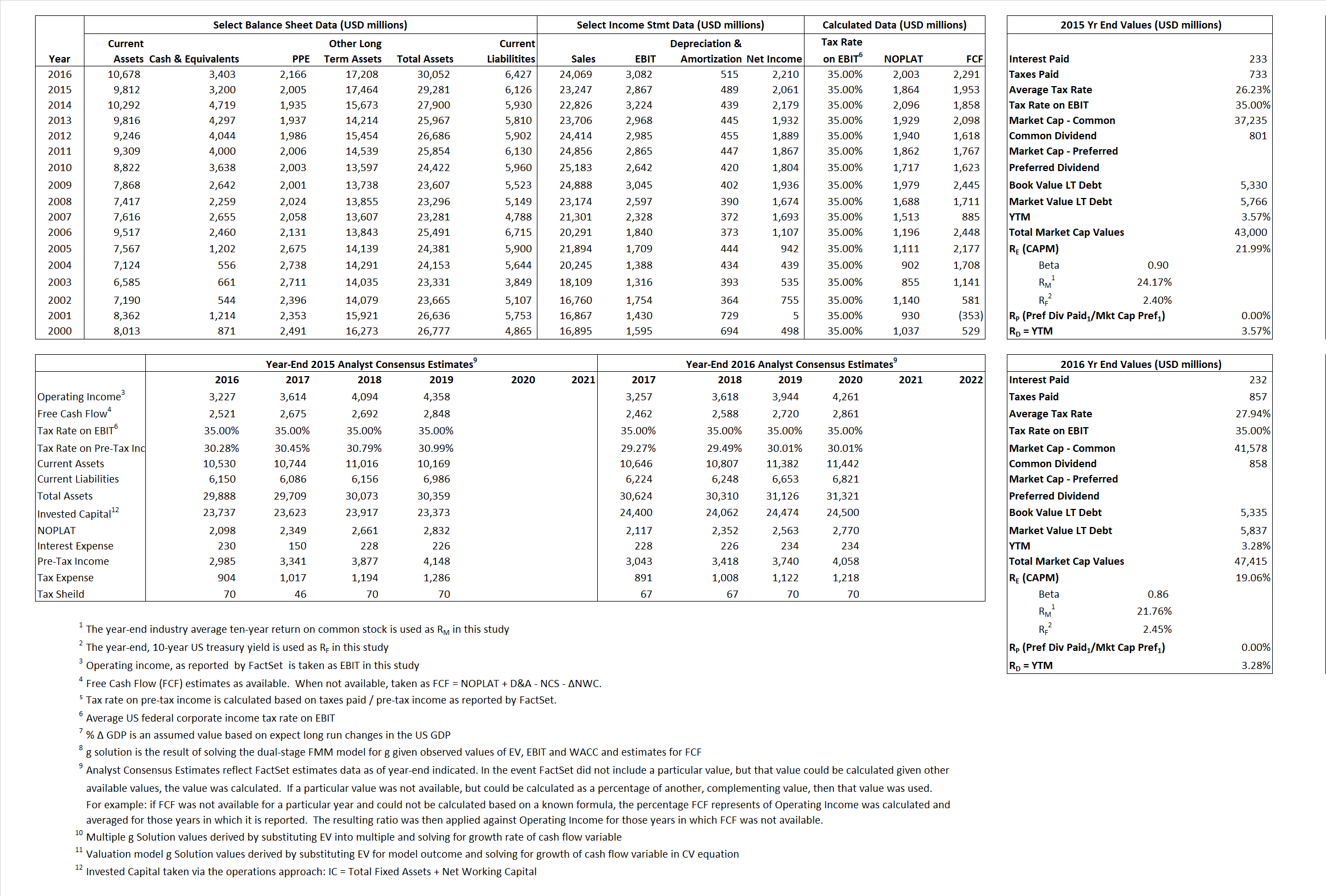# Raytheon Company

## Analyst Listing

The following analysts provide coverage for the subject firm as of May 2016:

 Broker Analyst Analyst Email Cowen & Company Cai Von Rumohr cai.von-rumohr@cowen.com Bernstein Research Douglas S. Harned douglas.harned@bernstein.com Jefferies Howard A. Rubel hrubel@jefferies.com Wolfe Research Hunter K. Keay hkeay@wolferesearch.com Stifel Nicolaus Jospeh W. DeNardi denardij@stifel.com Deutsche Bank Research Myles Walton myles.walton@db.com CRT Capital Group Peter Arment parment@sterneageecrt.com Drexel Hamilton Peteral Skibitski pskibitski@drexelhamilton.com Buckingham Research Richard Safran rsafran@buckresearch.com Credit Suisse Robert Spingarn robert.spingarn@credit-suisse.com RBC Capital Markets Robert Stallard robert.stallard@rbccm.com Guggenheim Securities Roman Schwizer roman.schweizer@guggenheimpartners.com Wells Fargo Securties Sam J. Pearlstein sam.pearlstein@wellsfargo.com

## Primary Input Data## Derived Input Data

### Equational Form

Net Operating Profit Less Adjusted Taxes NOPLAT  1,864 2,003$NOPLAT\, =\, EBIT\, x\, (1 \,-\, Avg \,\,Tax\,\, Rate\,\, on\,\, EBIT)$
Free Cash Flow FCF 1,953 2,291$FCF\,=NOPLAT\,+\,Non-Cash\,Expenses-\Delta NWC\,-\,NCS$
Tax Shield TS 61 65$TS\,=\,Interest\,\,Paid\,\,x\,\, Avg \,\,Tax\,\,Rate\,\, on\,\, Pre-Tax\,\, Income$
Invested Capital IC 23,155 23,625$IC\,=\,Fixed\,\,Operating\,\,Assets\,\,+\,\,Net\,\, Working\,\, Capital$
Return on Invested Capital ROIC 8.05% 8.48%$ROIC\,=\,\frac { NOPLAT }{ IC }$
Net Investment NetInv 1,674 985$NetInv\,=\,{ {IC}_{1}}-{{IC}_{0}}+Depreciation$
Investment Rate IR  89.83%  49.17%$IR\,=\,\frac {NetInv}{NOPLAT}$
Weighted Average Cost of Capital
WACCMarket 19.40%  17.00%$WACC\,=\,\frac { E }{ V } { R }_{ E }\,+\,\frac { P }{ V } { R }_{ P }\,+\,\frac { D }{ V } { R }_{ D }\left( 1- Avg\,\, Tax\,\,Rate\,\,on\,\,Pre-Tax\,\,Income \right)$
WACCBook  7.86%   7.37%
Enterprise value
EVMarket 39,800 44,012$EV\,=\,Market\,\,Cap\,\,Equity\,+\,\,Long\,\,Term\,\,Debt\,-\,Cash$
EVBook  39,162  43,510
Long-Run Growth
g = IR x ROIC
7.23%   4.17% Long-run growth rates of the income variable are used in the Continuing Value portion of the valuation models.
g = %$\Delta$ GDP    2.50%   2.50%
Margin from Operations M  12.33%  12.80%$M\,\,=\,\,\frac{EBIT}{SALES}$
Depreciation/Amortization Rate D  14.57%  14.32%$D\,\,=\,\,\frac{D+A}{EBITDA}$

## Valuation Multiple Outcomes

The outcomes presented in this study are the result of original input data, derived data, and synthesized inputs.

### model g solution

12/31/2015 12/31/2016 12/31/2015 12/31/2016 12/31/2015 12/31/2016

EV/SALES$\frac {EV}{Sales} \,= \,\frac{ROIC\, -\, g}{ROIC\,(WACC\,-\,g)}\,(1\,-\,T)\,(M)$

1.71 1.83 35.18% 26.88%  25.43%  20.42%

EV/EBITDA$\frac {EV}{EBITDA} \,= \,\frac{ROIC\, -\, g}{ROIC\,(WACC\,-\,g)}\,(1\,-\,T)\,(1\,-\,D)$

11.86 12.24 35.18% 26.88% 25.43% 20.42%

EV/NOPLAT$\frac {EV}{NOPLAT} \,= \,\frac{ROIC\, -\, g}{ROIC\,(WACC\,-\,g)}$

21.36 21.97 35.18% 26.88% 25.43% 20.42%

EV/FCFOPS$\frac {EV}{FCF_{OPS}} \,= \,\frac{ROIC\, -\, g}{ROIC\,(WACC\,-\,g)}\,(1\,-\,T)$

20.38 19.21 35.18% 26.88% 25.43% 20.42%

EV/EBIT$\frac {EV}{EBIT} \,= \,\frac{ROIC\, -\, g}{ROIC\,(WACC\,-\,g)}\,(1\,-\,T)$

13.88 14.28 35.18% 26.88% 25.43% 20.42%

EV/IC$\frac {EV}{IC} \,= \,\frac{ROIC\, -\, g}{WACC\,-\,g}$

1.72 1.86 35.18% 26.88% 25.43% 20.42%